Perimeter

## Perimeter and Circumference.

The distance around a shape is called its perimeter.

The perimeter of a circle is its circumference.

### How to get the Perimeter of plain shapes

#### 1. Rectangle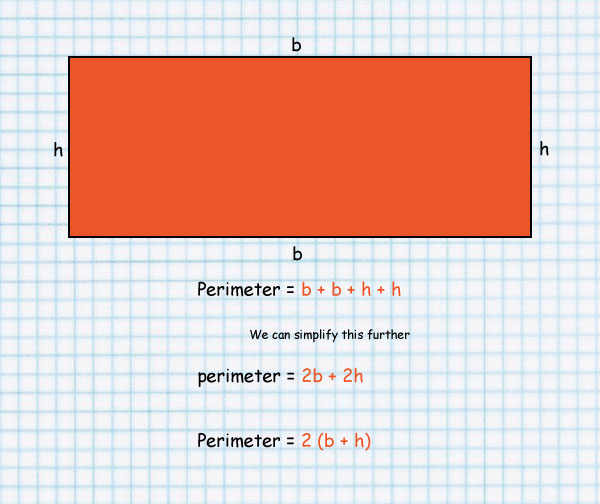#### 2. Square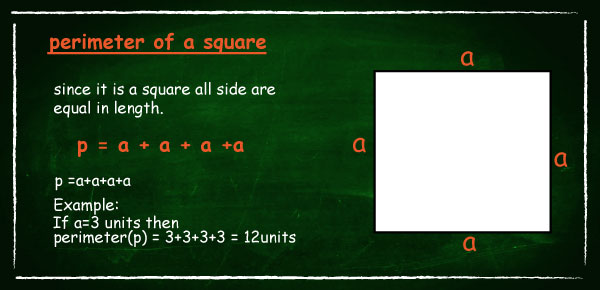#### 3. Full circle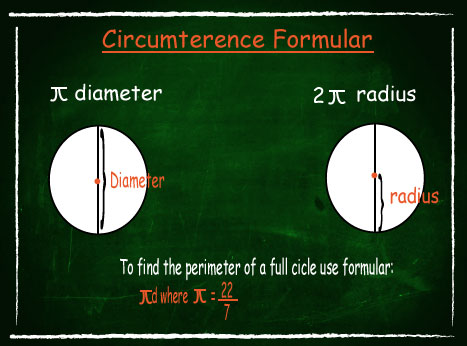#### 4. Semi circle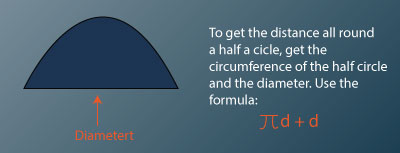• To find the perimeter of a plain shape like a rectangle, use the formula 2(L + W)
• To find the perimeter of a square use 4 X L
• To get the circumference of a full circle use πd
• To get the distance all round a half a circle, get the circumference of the half circle and the diameter. Use the formula πd + d
• To get the distance all round a figure made up of various shapes then add up the measurements.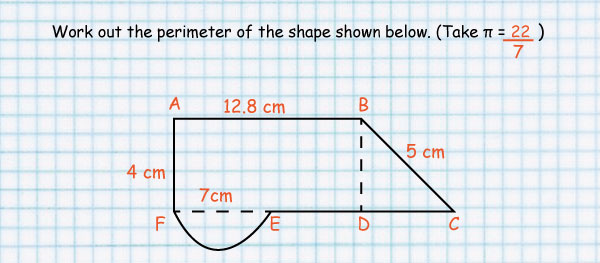## KCPE Topical Questions

Class 7

### Perimeter and Circumference

1. The figure below is a semi-circle of diameter 14 metres. What is the perimeter of the figure? (Take pi - 22/7).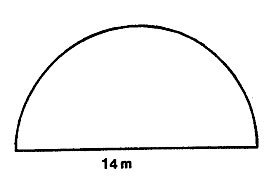A. 77 m     B. 36 m     C. 22 m        D. 58 m

2. Wanyama wants to put a fence round his rectangular plot 36 m long and 30 m wide. The interval between the posts is 3 m and the cost of each fencing post is sh 10.

Calculate the amount of money Wanyama will require to buy fencing posts.

A. Sh 450       B. Sh 220         C. Sh 440       D. Sh 3600

3. In the figure below, the diameter of the semicircle is 21 cm. What is the perimeter of the figure in centimeters? (Take 22/7)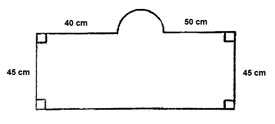A. 335       B. 323      C. 290       D. 302

4. The diagram below represents Ojil’s piece of land. He put a fence around it. How many posts did he use if the spacing between the posts was 3 metres?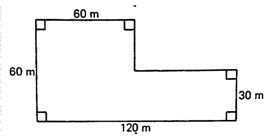A. 120      B. 90      C. 360      D. 121

5. The figure below represents a flower garden. The diameter PQRS of the larger semicircle is 28 m. the diameter QR of the smaller semicircle is 14 m.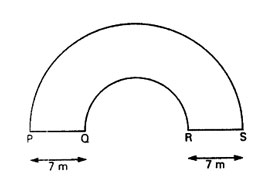What is the perimeter of this garden?

A. 66 m      B. 80 m      C. 146 m       D. 231 m

6. A rectangular plot of land length 44 m has an area of 1760 m2. What is the perimeter of this plot of land?

A. 84 m       B. 128 m       C. 176 m        D. 168 m

7. A bicycle wheel has a radius of 35 cm. What distance, in kilometres, does the wheel cover the wheel after 100 revolutions? (Take pi - 22/7)

A. 0.22       B. 2.2        C. 22          D. 220

8. Mwaka used 44 pegs to mark around a circular animal shed. The interval between the pegs was 3 m. What is the radius of the shed? (Take pi - 22/7)

A. 132 m       B. 42 m        C. 7 m          D. 21 m

9. The diagram below shows Amalemba’s piece of land. He put a fence around it.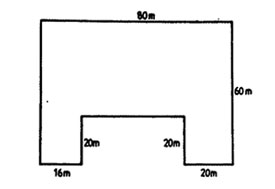How many posts did he use if the spacing between the posts was 4 metres?

A. 54       B. 79       C. 80        D. 81

10. A wheel covers a distance of 44 metres after making 10 revolutions. What is the radius of the wheel in metres? (Take pi - 22/7)

A. 0.7 m        B. 1.4 m        C. 2.2 m      D. 7.0 m

11. The diagram below shows Wanja’s plot of land.What is the perimeter of the plot?

A. 300       B. 280        C. 270           D. 230

12. The diagram below represents a vegetable garden. One of its edges is a semi-circle.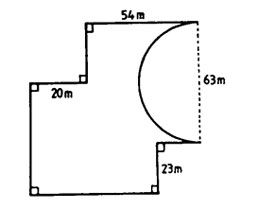What is the perimeter of the garden? (Take pi - 22/7)

A. 455 m     B. 356 m       C. 320 m       D. 160 m

13. The diagram below shows a running track whose dimensions are given in metres. The end of the track are semi-circular.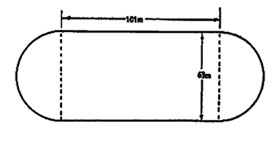What is the perimeter of the track? (Take pi - 22/7)

A. 198 m       B. 301 m       C. 328 m      D. 400 m

14. The figure below represents a plot surrounded by a part of a circular path and two straight paths. The two straight paths, each measuring 6.3 m, are also radii of the circle meeting at a right angle.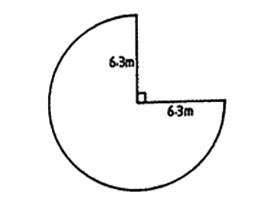What is the total length of the path surrounding the plot? (Take pi - 22/7)

A. 12.6 m       B. 29.7 m      C. 39.6 m     D. 42.3 m

15. The length of a side of a square plot of land is 26 m. A farmer left a space of 2 m for the gate and fenced round the remaining part with 3 strands of wire. What was the total length of the wire used?

A. 306 m     B. 310 m      C. 102 m      D. 312 m

16. A design was made of straight edges of lengths 2.1 cm and arcs of circles of radii 2.1 cm as shown in the diagram below.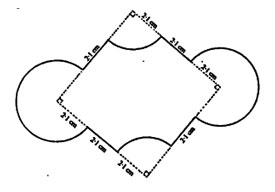What is the perimeter of the design? (Take pi - 22/7)

A. 36.12 cm     B. 34.8 cm      C. 26.4 cm       D. 21.6 cm

17. The figure below represents a vegetable garden.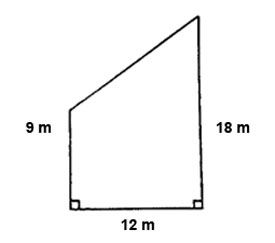What is the perimeter of the garden?

A. 39 m       B. 51 m       C. 54 m        D. 60 m

18. The design below is made up of a pair of parallel lines each 3 cm long, two small half-circles each of diameter 7 cm and two large half circles each of diameter 14 cm.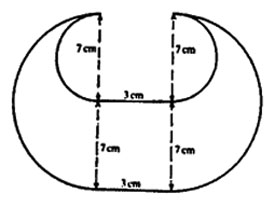What is the perimeter of the design? (Take pi - 22/7)

A. 39 cm       B. 66 cm      C. 72 cm       D. 136.5 cm

19. The figure below represents a plot of land.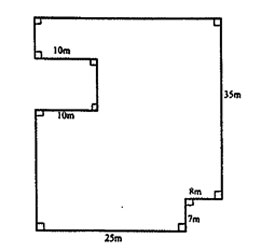How many poles, one metre apart, will be needed to fence the plot?

A. 171        B. 170        C. 169          D. 150

### Sources

• M4.2.1_perimeter by Unknown used under Creative Commons Attribution-NonCommercial-NoDerivatives 4.0 International License
• a3d0576b-2057-4473-9880-b5e1b801ed36 by elimu used under CC_BY-SA

elimu

used under CC_BY-SA
• perimeter_img1 by

elimu

used under CC_BY-SA
• perimeter_img2 by elimu used under CC_BY-SA
• perimeter_img3 by elimu used under CC_BY-SA
• 77c2ffa1-431a-4e82-b1b8-abf92181733d by elimu used under CC_BY-SA
• 4a1ded46-4d01-4332-849c-85eab2ad2bbc by elimu used under CC_BY-SA
• 7d3b152d-92c2-4884-a651-22d4739c6d69 by e-Limu used under CC_BY-SA
• a52f1f75-d2e2-48f8-adf3-5a384a207c00 by e-Limu used under CC_BY-SA
• bd6f3447-292f-4af4-8b3f-d2a89959f82f by e-Limu used under CC_BY-SA
• dad6b795-0317-4b07-8f74-c9de0c8230b1 by e-Limu used under CC_BY-SA
• f46ba850-c60b-4e38-aa81-7da6fe1eb814 by e-Limu used under CC_BY-SA
• 107c276b-d6e0-4ed7-acab-24c3186e0073 by e-Limu used under CC_BY-SA
• 1c965188-7829-462f-b068-562fa7e3019e by e-Limu used under CC_BY-SA
• 5cdd654b-270e-4bd8-bbd2-c75155abcda6 by e-Limu used under CC_BY-SA
• 5d7ce2b4-e89e-46c9-a452-392860e27fb8 by e-Limu used under CC_BY-SA
• 61b7c383-5a38-447d-aafd-915d45cdcefa by e-Limu used under CC_BY-SA
• 8b1ffdd1-a54f-4369-a597-37bb1b9e4ebf by e-Limu used under CC_BY-SA
• e7e02a15-4431-451a-b285-c87d6516f5c9 by e-Limu used under CC_BY-SA
• ecc98b16-dc7f-4ef0-acfd-71bfe2dacfc0 by e-Limu used under CC_BY-SA

•